# CIE A Level Maths: Mechanics复习笔记1.1.4 Types of Force

### Types of Force

#### What is a force?

• A force is a vector quantity, it has both magnitude and direction
• A force is a push or a pull on an object
• A force is measured in Newtons
• In A level mathematical models, forces act at a single point called a particle which occupies a single point in space

#### What types of force are used in mechanics?

• Weight is the effect of mass and gravity, it always acts downwards
• Tension is a pulling force, it always acts away from an object
• Thrust is a pushing force, it always acts towards an object
• Friction is a resistive force, it acts to oppose the motion of an object
• Every surface will produce a reaction force, it will always act perpendicular to the surface

#### Mass, Gravity and Weight

• Mass is a measure of the amount of matter in an object
• Mass is a scalar quantity, measured in kilogramskg
• Mass is universal, it does not change based on location
• Gravity is the force by which a body (usually a planet or a star) pulls objects towards its centre
• is the acceleration due to gravity, measured in m s-2
• On Earth, is approximately 10 m s-2 , although its exact value varies with location
• is different elsewhere in the universe
• Weight is the product of mass and gravity, it is a force measured in Newtons
• Weight is a vector quantity
• Weight varies with location
• Weight always acts vertically downwards towards the ground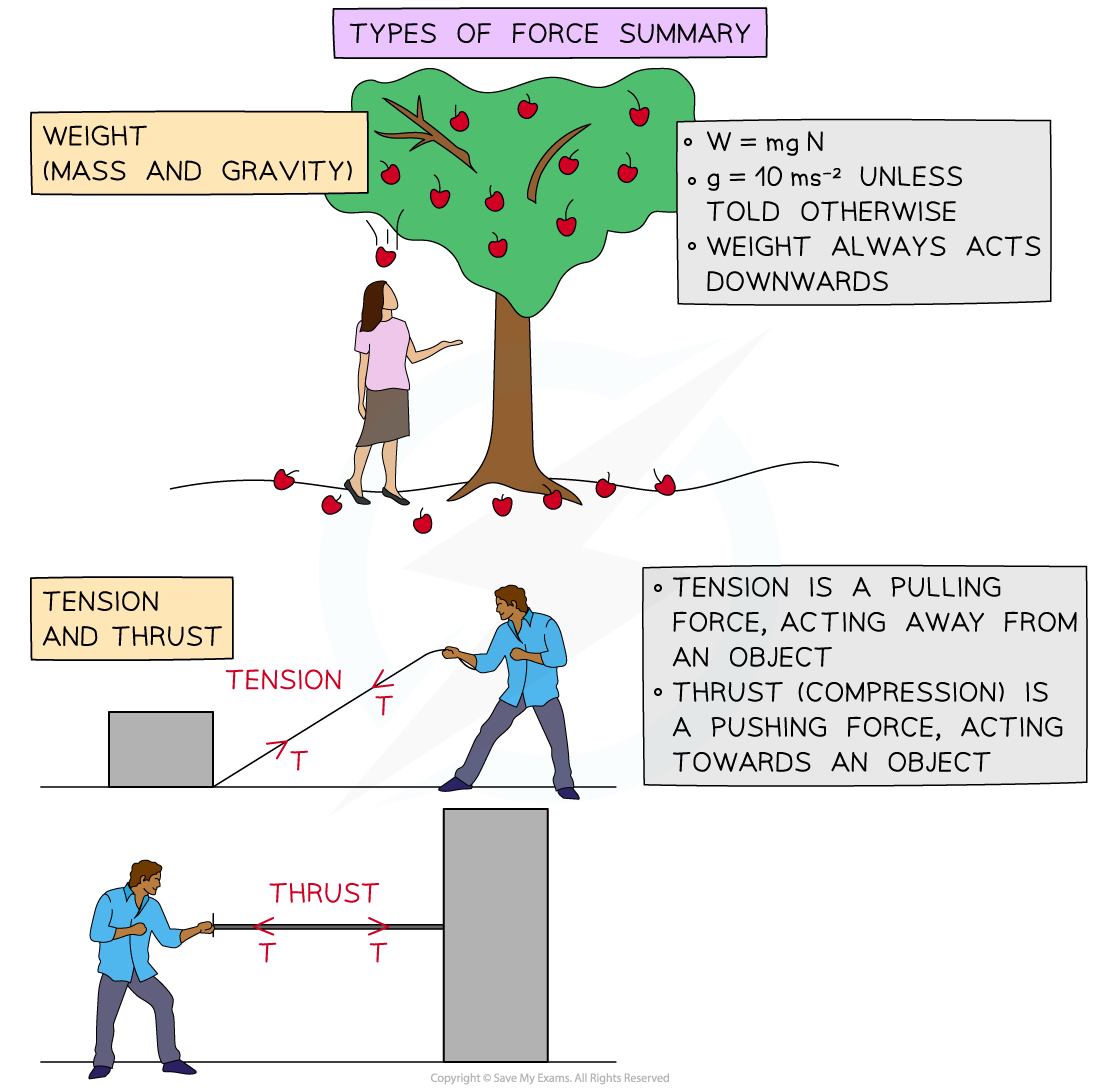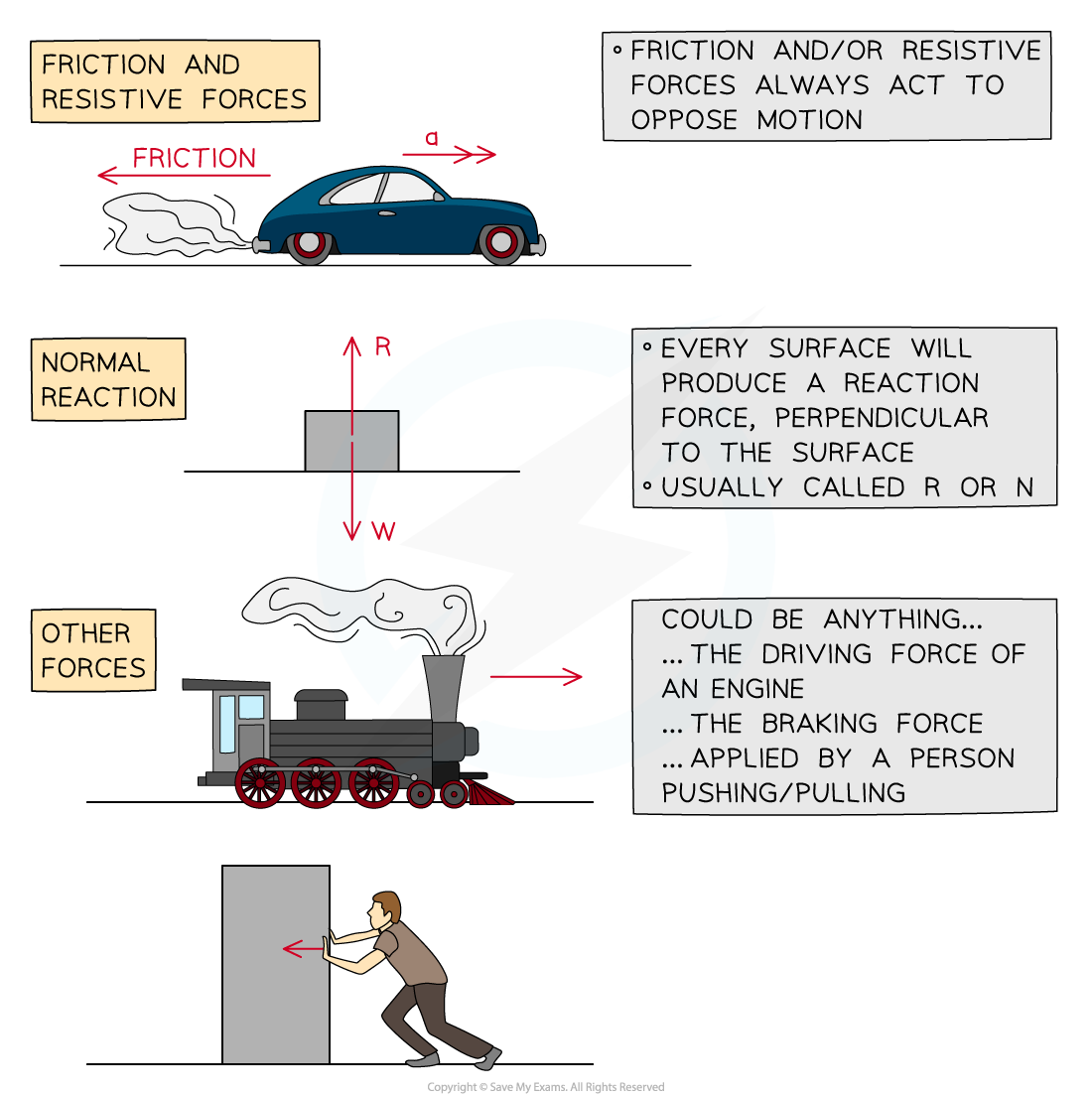#### Worked Example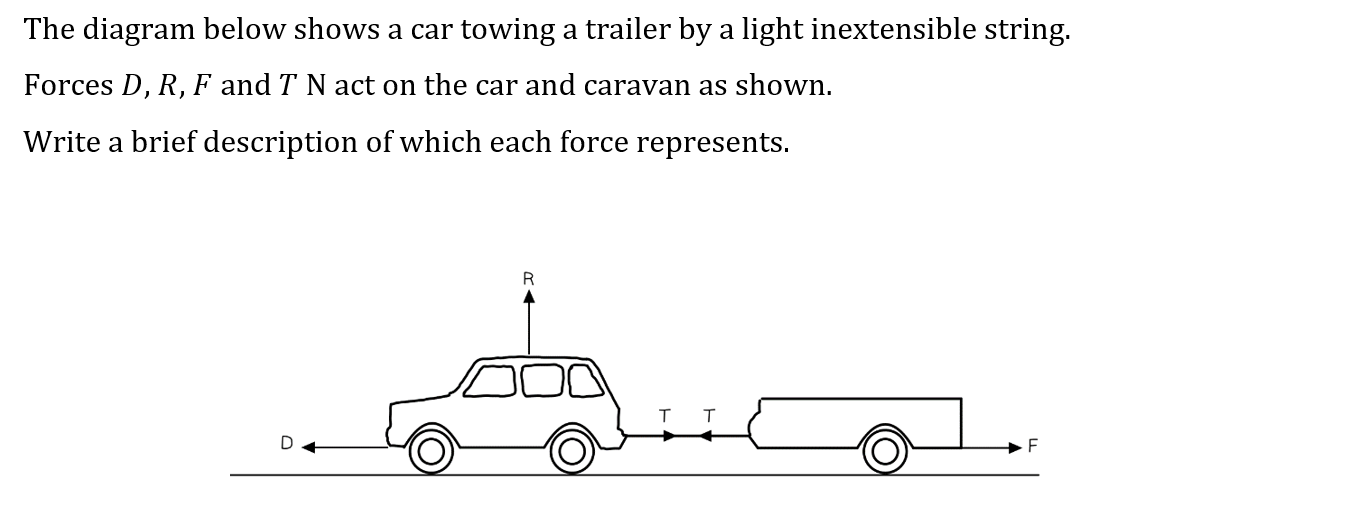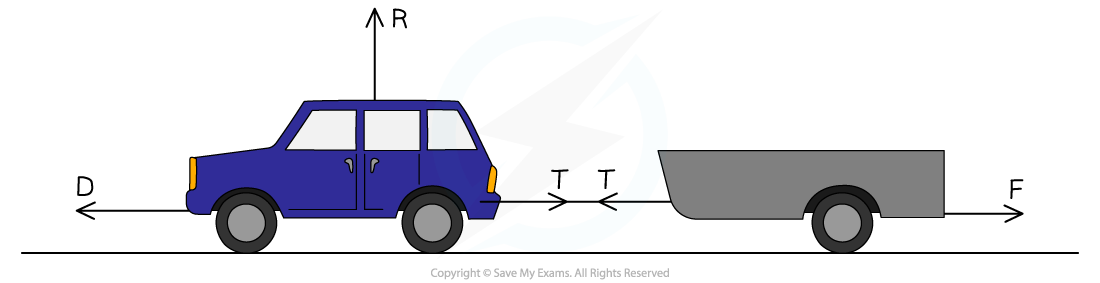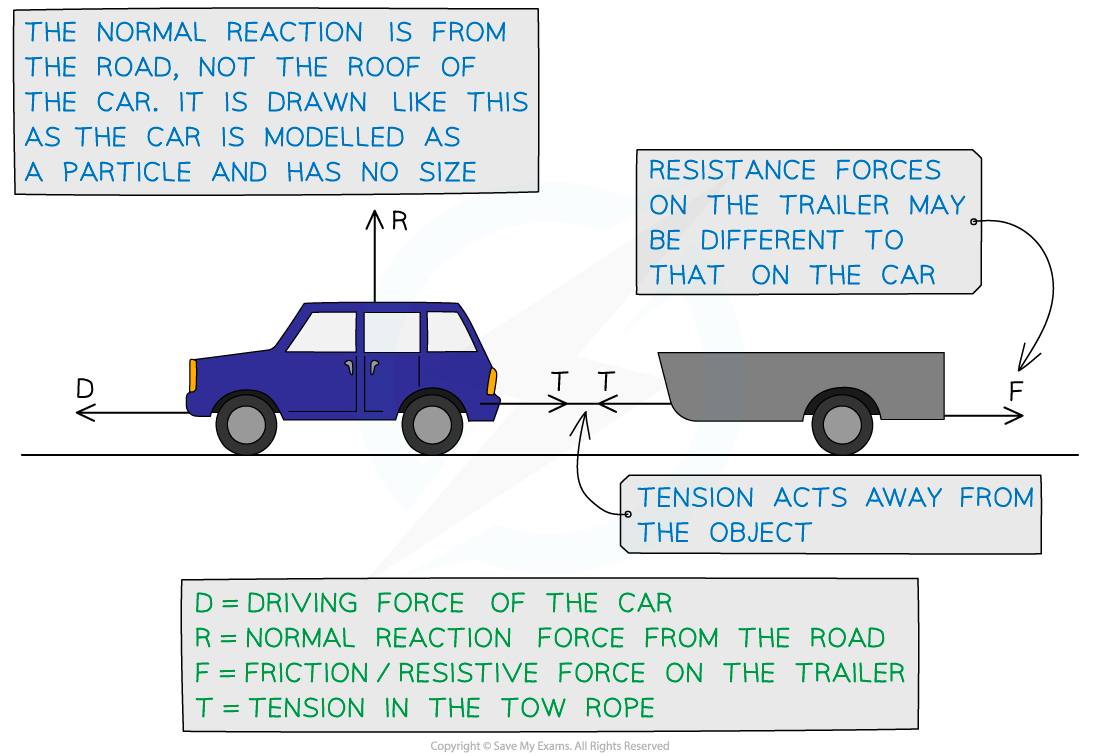#### Exam Tip

• Always sketch a diagram and mark the forces on the diagram clearly, it will help you to understand the problem. Add more things to the diagram as you progress through the question. You may not even need all the forces from your diagram but it is useful to have them there.
• You should always round your answers to three significant figures.